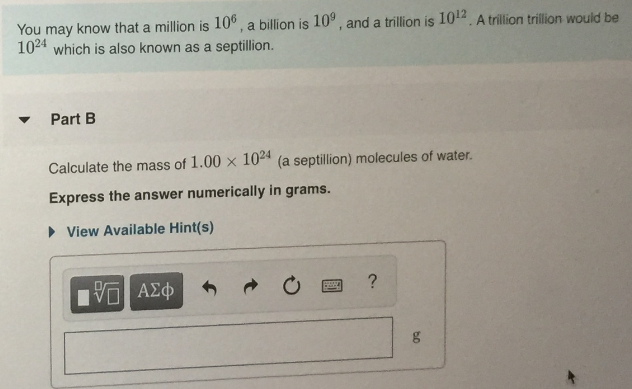# You may know that a million is 10^6, a billion is 10^9, and a trillion is 10^12. A trillion trillion would be 110^24 which is known as a septillion. Calculate the mass of 1.00 x 10^24 (a septiliion) molecules of water. Express the answer numerically in grams.